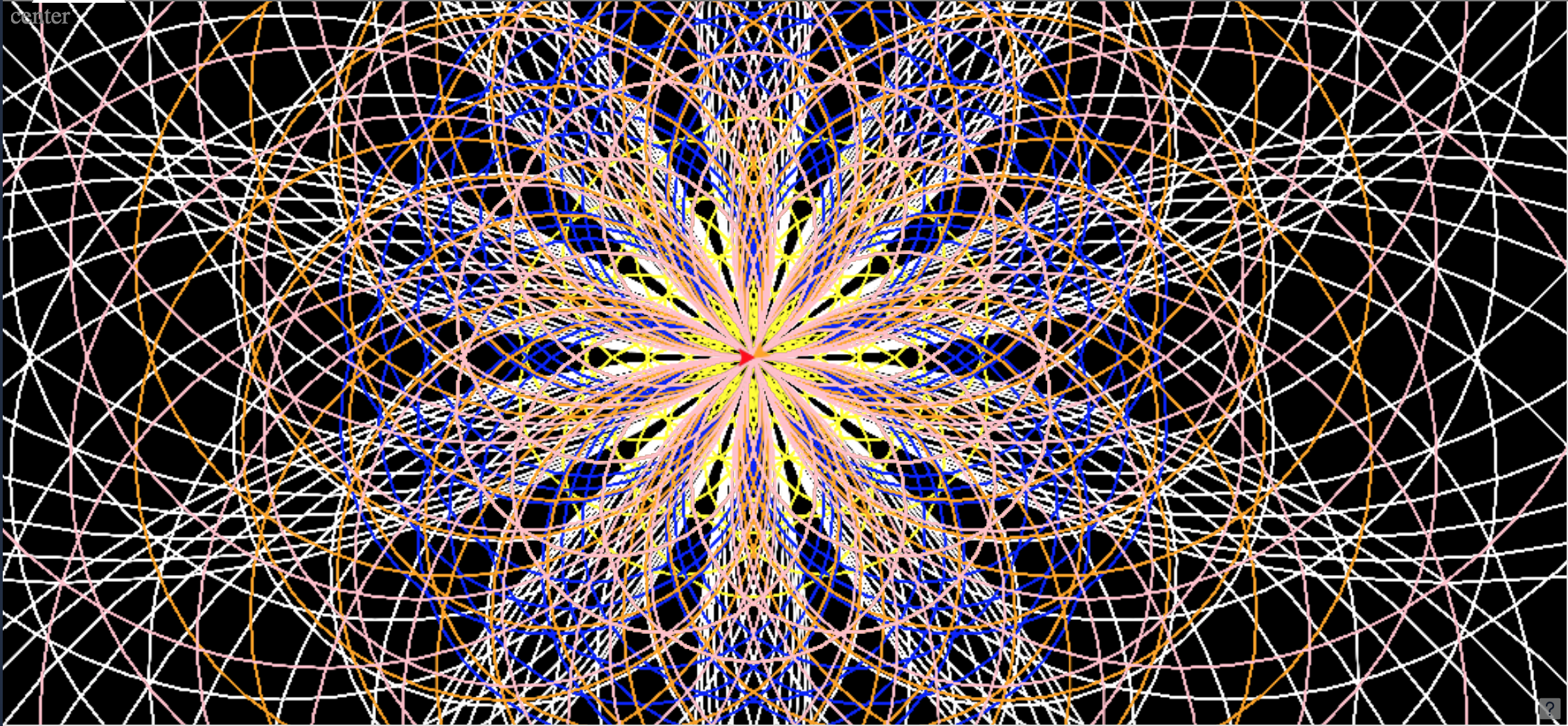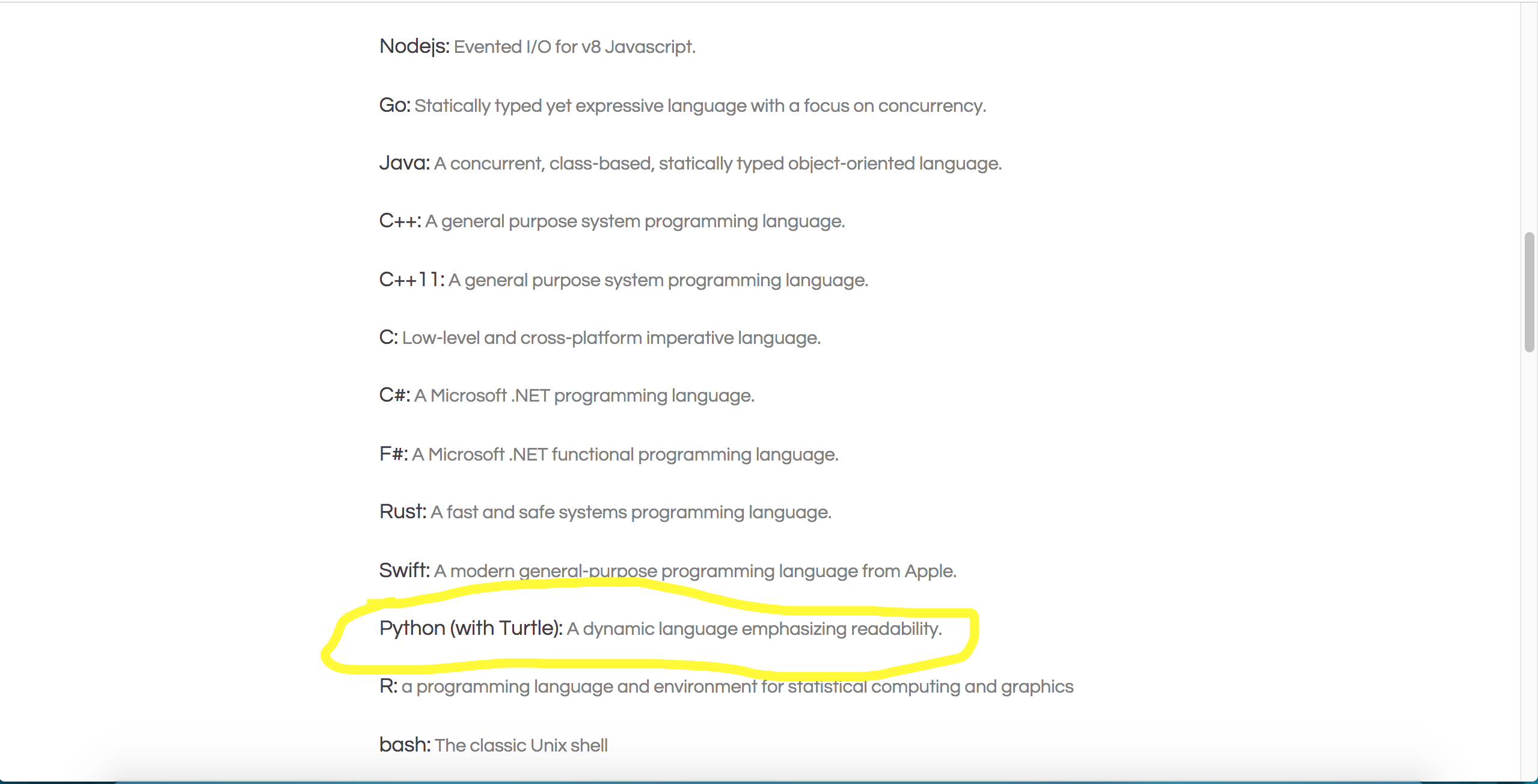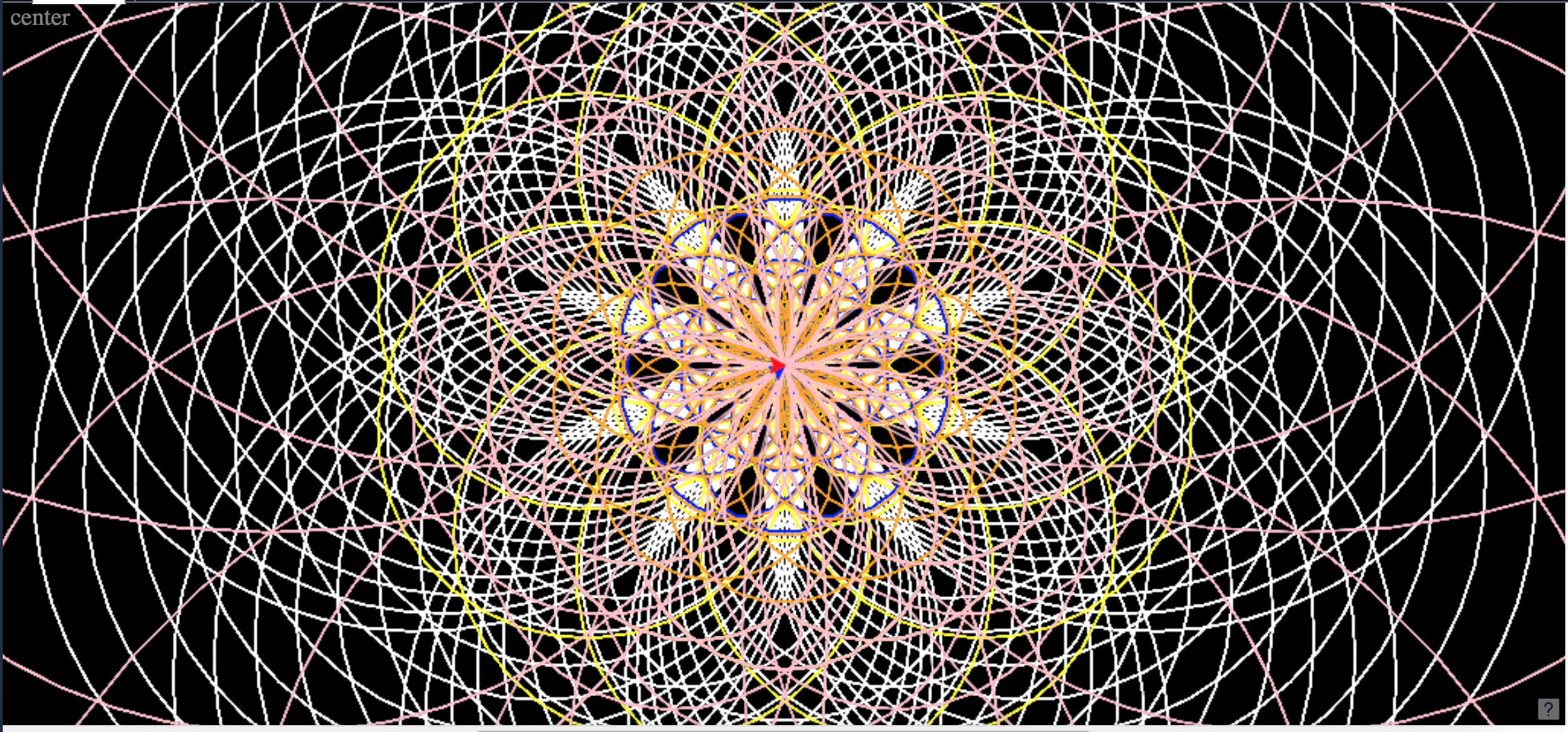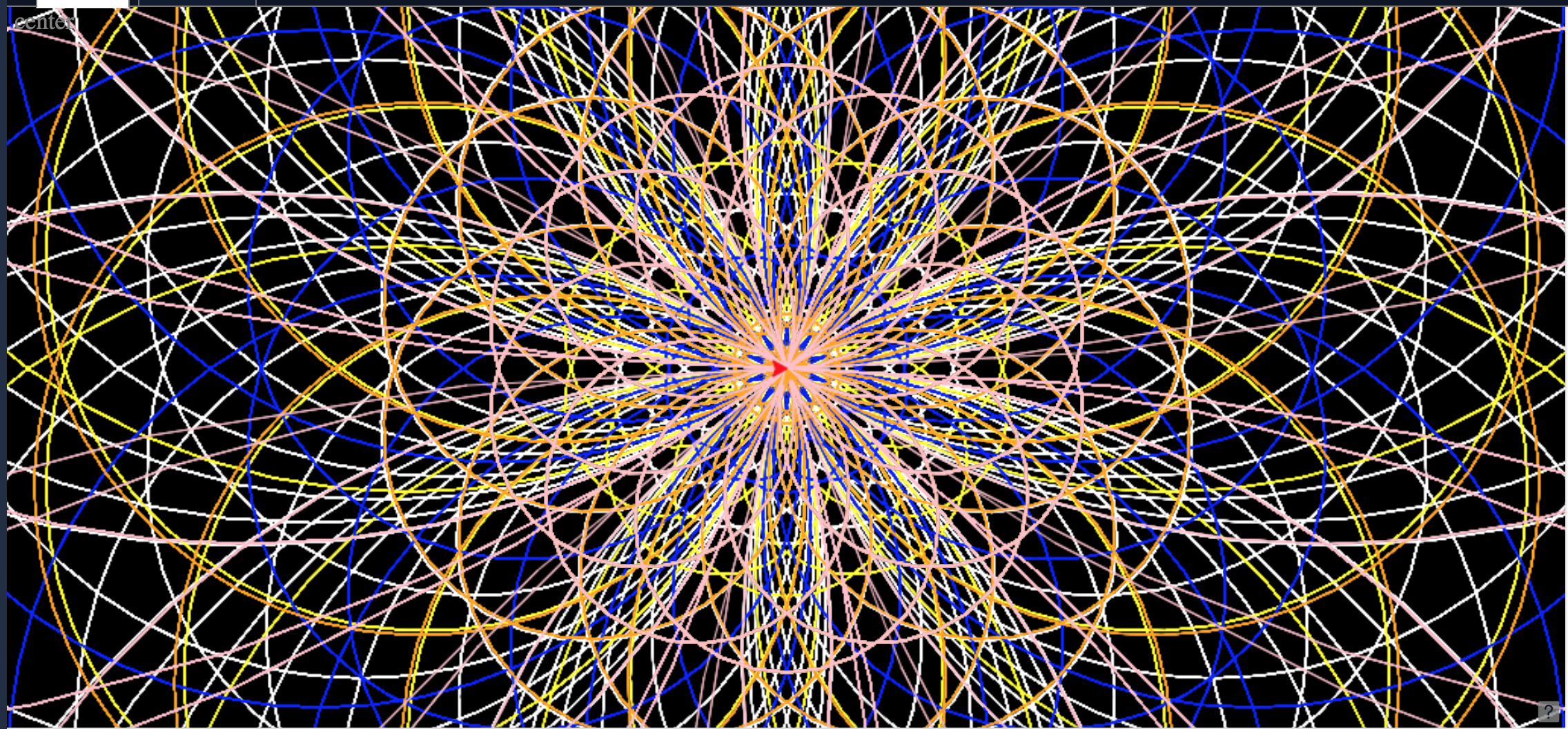Colorful Circle Spiral Generator Using Python Turtle
[deleted]

Welcome to the python turtle circle pattern tutorial! In this tutorial you will learn how to make a colorful circle pattern creator with python using turtle! This patterns will look somewhat like this and the best part is that you can customize them!First log on to your repl.it account and make a new python turtle codeOn your new repl.it first you are going to put in your imports. For this code you will need to import math, and obviously turtle. It should look like this now!

``````import turtle
import math ``````

We now want to set the background color! To do this you are going to type

``````turtle.Screen().bgcolor('black')
#This sets the color to black but you can choose any background color! ``````

Now you need to create 5 turtles for this code. I named mine Johny, Steve, Terry, Barry, ,Will, and Nich yours can be named anything you would like. Just make sure to use the same name every time. I also defined its speed and color!

``````Johny  =  turtle.Turtle()
Johny.speed(0)
Johny.color('white')
#When the speed of the turtle is set to 0 then it will turn off the animation and it will go as fast as possible. I used the color white for my turtle but you can use any color you want it does not matter :). Do this 5 times for 5 different names and 5 different colors but keep the speed the same!``````

So far our code looks like this:

``````import turtle
import math

turtle.Screen().bgcolor('black')
Johny  =  turtle.Turtle()
Johny.speed(0)
Johny.color('white')

Steve = turtle.Turtle()
Steve.speed(0)
Steve.color('yellow')

Barry = turtle.Turtle()
Barry.speed(0)
Barry.color('blue')

Terry = turtle.Turtle()
Terry.speed(0)
Terry.color('orange')

Will = turtle.Turtle()
Will.speed(0)
Will.color('pink')

Nich = turtle.Turtle()
Nich.speed(0)
Nich.color('red')
#When the speed of the turtle is set to 0 then it will turn off the animation and it will go as fast as possible``````

To make this colorful circle generator you would want a user input so that you can get a new pattern each time!. To do this use a input statement and then a statement to make sure your number is a integer. It will look like this

``````random = input("Enter a random number")
random = int(random)``````

Now we will need to make a while true loop so that the pattern will keep drawing forever and this will look like this:

``while True:``

Anything which is in this while true loop needs to be tabbed in!

We are very close to finishing our code!

Inside this while true loop you will need to put this code in

``````def drawCircles(t,size):
for i in range(10):
t.circle(size)
size=size- minus+62
def drawSpecial(t,size,repeat):
for i in range (repeat):
drawCircles(t,size)
t.right(360/repeat)
drawSpecial(Johny ,100,10)``````

There are comments in the code to tell you what this code means. In this code the turtle Johny is drawing which means it will be the color white.

Now you will want to put this code in for each turtle and you will be done! I recommend that for every size = size - minus + 62 line that you change the +62 to something else like -12 or *32 to get a very interesting pattern each time.

Here is the final code.

``````import turtle
import math

turtle.Screen().bgcolor('black')

Johny = turtle.Turtle()
Johny.speed(0)
Johny.color('white')
Steve = turtle.Turtle()
Steve.speed(0)
Steve.color('yellow')
Barry = turtle.Turtle()
Barry.speed(0)
Barry.color('blue')
Terry = turtle.Turtle()
Terry.speed(0)
Terry.color('orange')
Will = turtle.Turtle()
Will.speed(0)
Will.color('pink')
Nich = turtle.Turtle()
Nich.speed(0)
Nich.color('red')

minus = input("Give me a random number")
minus = int(minus)
print "Click on results now"

while True:

def drawCircles(t,size):
for i in range(10):
t.circle(size)
size=size- minus+62
def drawSpecial(t,size,repeat):
for i in range (repeat):
drawCircles(t,size)
t.right(360/repeat)
drawSpecial(Johny,100,10)

def drawCircles(t,size):
for i in range(4):
t.circle(size)
size=size - minus -3
def drawSpecial(t,size,repeat):
for i in range (repeat):
drawCircles(t,size)
t.right(360/repeat)
drawSpecial(Steve,100,10)

def drawCircles(t,size):
for i in range(4):
t.circle(size)
size=size- minus
def drawSpecial(t,size,repeat):
for i in range (repeat):
drawCircles(t,size)
t.right(360/repeat)
drawSpecial(Barry,100,10)

def drawCircles(t,size):
for i in range(4):
t.circle(size)
size=size- minus + 2
def drawSpecial(t,size,repeat):
for i in range (repeat):
drawCircles(t,size)
t.right(360/repeat)
drawSpecial(Terry,100,10)

def drawCircles(t,size):
for i in range(4):
t.circle(size)
size=size-minus*3
def drawSpecial(t,size,repeat):
for i in range (repeat):
drawCircles(t,size)
t.right(360/repeat)
drawSpecial(Will,100,10)

def drawCircles(t,size):
for i in range(4):
t.circle(size)
size=size-minus-45

def drawSpecial(t,size,repeat):
for i in range (repeat):
drawCircles(t,size)
t.right(360/repeat)
drawSpecial(Nich,100,10)``````

To run the code you first must press run button and then you have to go to console and type your random number. Your random number can be a negative number and if you make the number too big the code does not work. After you do that then you should be good to go into result and view your pattern. If you discovered a cool looking pattern put it down below in the comments!

Here is are some cool patterns the generator made!Thanks to nothplus for the idea of making a tutorial for python turtle! Be sure to check his tutorial right here>>>https://repl.it/talk/challenge/How-To-Create-A-Black-Hole-Spiral-in-Python-W-Turtle/7423

# Stay Tuned For More! And make sure to smash that upvote button

You are viewing a single comment. View All
[deleted]

@AnkushAnkush Once you put the random number you need to go to the result tab.